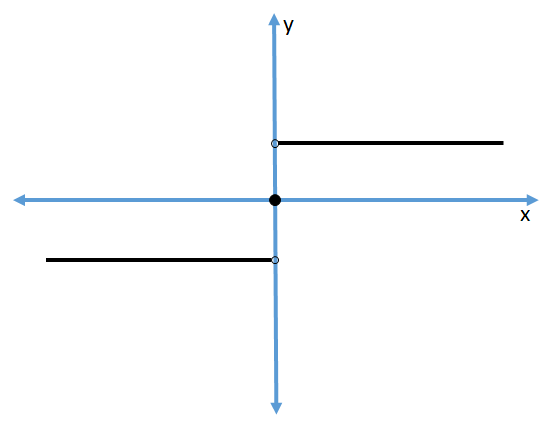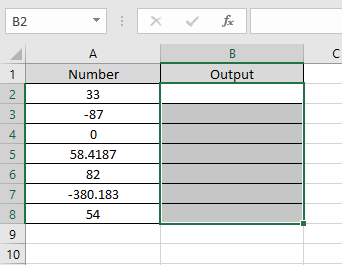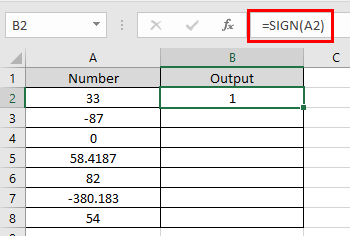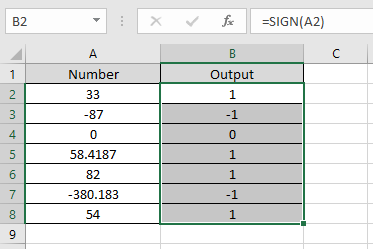# How to use the SIGN Function in Excel

SIGN function in excel is a mathematical function. In mathematics, it is known as signum function which returns -1 for all the negative numbers, 0 for only 0 number and +1 for all the positive numbers.

The graph for the SIGN function where x-axis represent all the input values and y-axis represent all the output values for the input values.The SIGN function checks the sign of number and returns -1 for input negative numbers, 0 for input 0 and +1 for the input positive number.
Syntax:

=SIGN (number)

Number : input number directly or via cell reference.
Let’s understand this function using it in an example.Here we have some values where we need to check whether the value is positive, negative or zero.
Use the formula:

=SIGN(A2

A2 : number
Here argument to the formula is taken as cell reference.As you can see, the formula returns 1 for the first value which is a positive number.

Now copy the formula to other cells using the shortcut key Ctrl + DSIGN function returns the sign of the input numbers. You can use the function with other excel functions to get results.

Note : The function generates #VALUE! Error, if the argument to the function is non - numeric.

Hope you understood how to use SIGN function and referring cell in Excel. Explore more articles on Excel function here. Please feel free to state your query or feedback for the above article.

Related Articles:

How to use the ABS Function in Excel

Excel Convert negative number to positive number

Popular Articles:

50 Excel Shortcuts to Increase Your Productivity

How to use the VLOOKUP Function in Excel

How to use the COUNTIF in Excel 2016

How to use the SUMIF Function in Excel

Terms and Conditions of use

The applications/code on this site are distributed as is and without warranties or liability. In no event shall the owner of the copyrights, or the authors of the applications/code be liable for any loss of profit, any problems or any damage resulting from the use or evaluation of the applications/code.